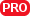#### File Exchange > Data Analysis >    Bayesian Ridge RegressionAuthor:
OriginLab Technical Support
7/2/2020
Last Update:
4/6/2021
127
Total Ratings:
0
File Size:
69 KB
Average Rating:File Name:
BRR.opx
File Version:
1.01
Minimum Versions:
Free
Summary:

Perform bayesian ridge regression with Python.

Screen Shot and Video:
Description:

Purpose

This App provides a tool for fitting data with Bayesian Ridge Regression model. It fits a dataset with one dependent variable and multiple independent variables. You can further use it to predict response of independent variables.

Notes:

• It needs Embedded Python and scikit-learn library. Other dependent libraries include numpy

Installation

1. Download the BRR.opx file, then drag-and-drop onto the Origin workspace.
2. The App will start downloading dependent Python libraries. Wait a few minutes until the download is completed and restart Origin.

Operation

1. Activate a worksheet. Click the App icon to bring up the dialog.
2. On Input Data tab, select single or multiple worksheet columns for Independent Variables and specify Dependent Variable by selecting a single worksheet column.
3. On Options tab, change settings to fit the model
• Maximum Iterations: Maximum number of iterations. The solver iterates until convergence or this number of iterations.
• Tolerance: Stop the algorithm if fitting parameter has converged.
• Alpha1: Shape parameter for the Gamma distribution prior over the alpha parameter.
• Alpha2: Inverse scale parameter (rate parameter) for the Gamma distribution prior over the alpha parameter.
• Lambda1: Shape parameter for the Gamma distribution prior over the lambda parameter.
• Lambda2: Inverse scale parameter (rate parameter) for the Gamma distribution prior over the lambda parameter.
• Alpha Initial: Initial value for alpha (precision of the noise). If not set, alpha_init is 1/Var(y).
• Lambda Initial: Initial value for lambda (precision of the weights). If not set, lambda_init is 1.
• Include Intercept: Whether to calculate the intercept for this model.
• Normalize: Whether to nomralize the dataset before regression.
4. On Quantities tab: choose which quantities and plots to output.
5. On Plot tab: choose plots to output.
• Score Plot:  Plot the log marginal likelihood as a function of iteration number.
6. On Prediction tab, you can select a range of independent data to predict the response with the fitted neural network.
7. Click OK to output reports.